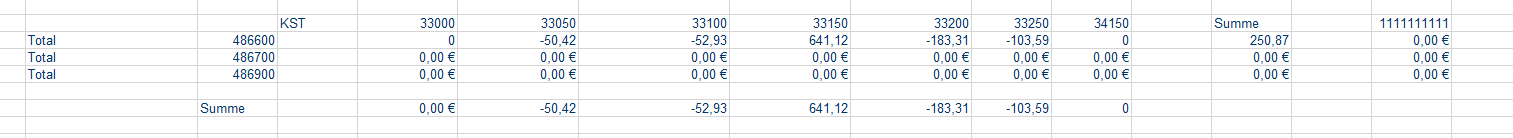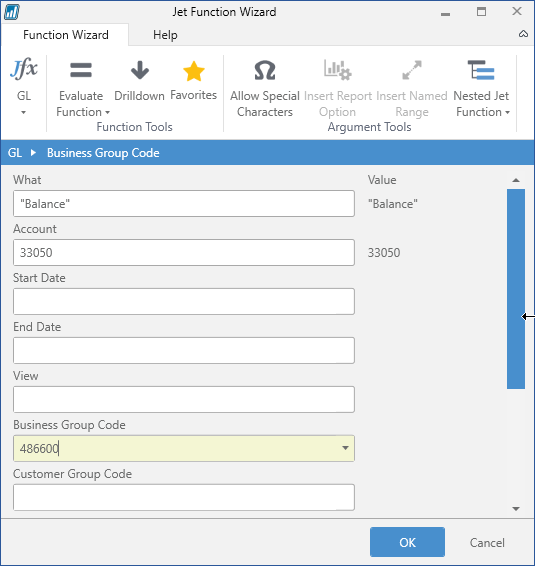Hi,

i try to build a matrix that has a totaling account from the chart of accounts in the rows and a totaling on cost-centers on the columns. Cost-centers are the global dimension 1. I always get zero as result.

Is it possible to build such a matrix with the GL Function?

Best Regards Christian

#### 3 comments

•Harry Lewis

Could you show us a picture of what you have in mind?

•ChrisThe 3 total rows are total accounts from the chart of accounts 486000, 487000, 487900.

The KST (cost center) are unique. 33000, 33050, ...

111111111 is a total of the 6 KST. This is a total from Navision in the Dimension values.

What i want to get per GL function is the result from two totals, e.g. 486400 and 111111111.

Is this possible?

Hope this helps to understand.

Thanks a lot.

•Harry Lewis

Thank you, Chris.

Your chart of accounts appears somewhat different than I have seen before, so I have a few more questions.

1) Does the value 111111111 represent the summation of the balance in accounts 33000, 33050, 33100, 33150, 33200, 33250, and 34150?

2) I assume that 486600, 486700, and 486900 are account numbers (for some totaling accounts).  Correct?

3) In the 33050 column, you have the value -50.42.  Is that the GL balance for account # 33050 with the Global Dimension #1 of 486600 applied?

like this (my Global Dimension 1 is "Business Group Code")?Please sign in to leave a comment.